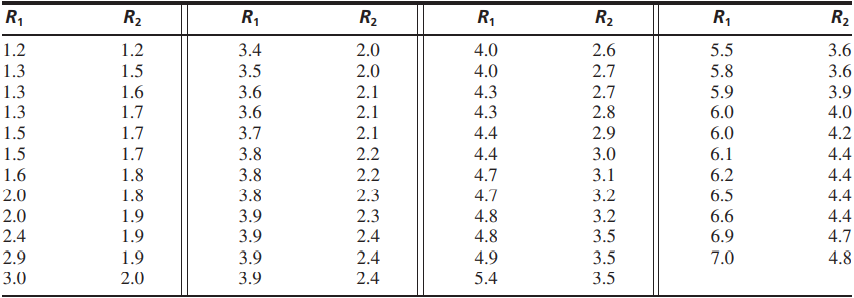×
Get Full Access to Statistics For Engineers And Scientists - 4 Edition - Chapter 7.4 - Problem 10e
Get Full Access to Statistics For Engineers And Scientists - 4 Edition - Chapter 7.4 - Problem 10e

×

# Two radon detectors were placed in different locations inISBN: 9780073401331 38

## Solution for problem 10E Chapter 7.4

Statistics for Engineers and Scientists | 4th Edition

• Textbook Solutions
• 2901 Step-by-step solutions solved by professors and subject experts
• Get 24/7 help from StudySoup virtual teaching assistantsStatistics for Engineers and Scientists | 4th Edition

4 5 1 311 Reviews
16
4
Problem 10E

Two radon detectors were placed in different locations in the basement of a home. Each provided an hourly measurement of the radon concentration, in units of pCi/L. The data are presented in the following table.a. Compute the least-squares line for predicting the radon concentration at location 2 from the concentration at location 1.

b. Plot the residuals versus the fitted values. Does the linear model seem appropriate?

c. Divide the data into two groups: points where R1 < 4 in one group, points where R1 ≥ 4 in the other. Compute the least-squares line and the residual plot for each group. Does the line describe either group well? Which one?

d. Explain why it might be a good idea to fit a linear model to part of these data, and a nonlinear model to the other.

Step-by-Step Solution:
Step 1 of 3

Step 2 of 3

Step 3 of 3

##### ISBN: 9780073401331

This textbook survival guide was created for the textbook: Statistics for Engineers and Scientists , edition: 4. This full solution covers the following key subjects: concentration, Group, line, model, data. This expansive textbook survival guide covers 153 chapters, and 2440 solutions. The answer to “?Two radon detectors were placed in different locations in the basement of a home. Each provided an hourly measurement of the radon concentration, in units of pCi/L. The data are presented in the following table. a. Compute the least-squares line for predicting the radon concentration at location 2 from the concentration at location 1.b. Plot the residuals versus the fitted values. Does the linear model seem appropriate?c. Divide the data into two groups: points where R1 < 4 in one group, points where R1 ? 4 in the other. Compute the least-squares line and the residual plot for each group. Does the line describe either group well? Which one?d. Explain why it might be a good idea to fit a linear model to part of these data, and a nonlinear model to the other.” is broken down into a number of easy to follow steps, and 134 words. Statistics for Engineers and Scientists was written by and is associated to the ISBN: 9780073401331. The full step-by-step solution to problem: 10E from chapter: 7.4 was answered by , our top Statistics solution expert on 06/28/17, 11:15AM. Since the solution to 10E from 7.4 chapter was answered, more than 278 students have viewed the full step-by-step answer.

Unlock Textbook Solution